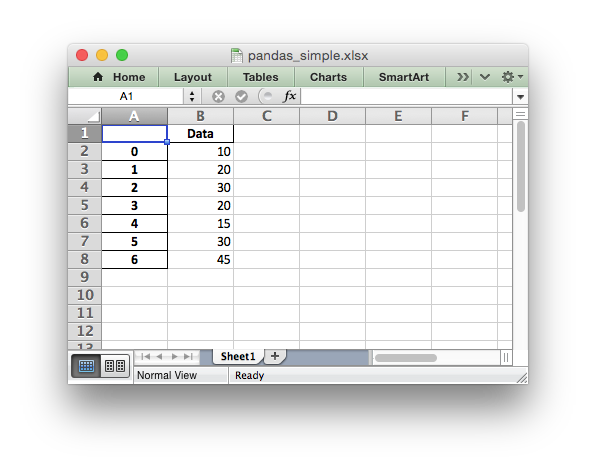# Example: Pandas Excel example

A simple example of converting a Pandas dataframe to an Excel file using Pandas and XlsxWriter. See Working with Pandas and XlsxWriter for more details.```##############################################################################
#
# A simple example of converting a Pandas dataframe to an xlsx file using
# Pandas and XlsxWriter.
#
# Copyright 2013-2023, John McNamara, jmcnamara@cpan.org
#

import pandas as pd

# Create a Pandas dataframe from some data.
df = pd.DataFrame({"Data": [10, 20, 30, 20, 15, 30, 45]})

# Create a Pandas Excel writer using XlsxWriter as the engine.
writer = pd.ExcelWriter("pandas_simple.xlsx", engine="xlsxwriter")

# Convert the dataframe to an XlsxWriter Excel object.
df.to_excel(writer, sheet_name="Sheet1")

# Close the Pandas Excel writer and output the Excel file.
writer.close()
```